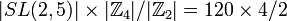# Central product of SL(2,5) and Z4

View a complete list of particular groups (this is a very huge list!)[SHOW MORE]

## Definition

This group is defined in the following equivalent ways:

1. It is the unique subgroup of index two inside general linear group:GL(2,5). Explicitly, it is the subgroup of general linear group:GL(2,5) comprising those matrices whose determinant is a square in field:F5 (which in this case means the determinant is$\pm 1$.
2. It is the central product of special linear group:SL(2,5) and cyclic group:Z4 with shared central subgroup cyclic group:Z2 (this is center of special linear group:SL(2,5) in SL(2,5) and Z2 in Z4 in cyclic group:Z4).

In symbols, the group is:$SL(2,5) *_{\mathbb{Z}_2} \mathbb{Z}_4$

## Arithmetic functions

Want to compare and contrast arithmetic function values with other groups of the same order? Check out groups of order 240#Arithmetic functions
Function Value Similar groups Explanation
order (number of elements, equivalently, cardinality or size of underlying set) 240 groups with same order product formula: the order is$|SL(2,5)| \times |\mathbb{Z}_4|/|\mathbb{Z}_2| = 120 \times 4/2$.

## GAP implementation

### Group ID

This finite group has order 240 and has ID 93 among the groups of order 240 in GAP's SmallGroup library. For context, there are 208 groups of order 240. It can thus be defined using GAP's SmallGroup function as:

SmallGroup(240,93)

For instance, we can use the following assignment in GAP to create the group and name it$G$:

gap> G := SmallGroup(240,93);

Conversely, to check whether a given group$G$ is in fact the group we want, we can use GAP's IdGroup function:

IdGroup(G) = [240,93]

or just do:

IdGroup(G)

to have GAP output the group ID, that we can then compare to what we want.

### Other descriptions

Description Functions used
MaximalNormalSubgroups(GL(2,5)) MaximalNormalSubgroups, GL